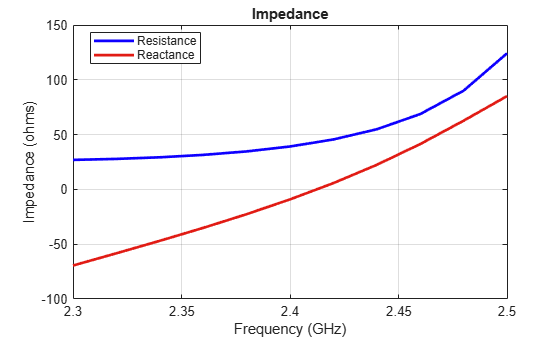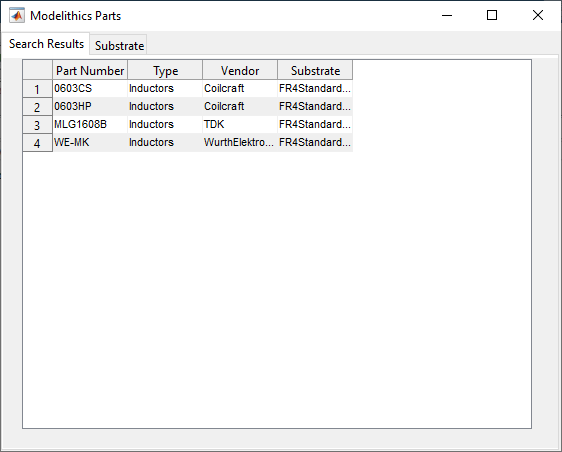# Design Matching Network Using Lumped Components from Modelithics Library

This example shows you how to design a matching network with real-world lumped components from Modelithics SELECT+ Library™. The example also shows you how to analyze this matching network by matching it to a reference antenna and comparing its performance to a matching network with ideal lumped elements. The reference antenna in the example is an inset-fed microstrip patch antenna with an operating frequency of 2.4 GHz. This allows you to consider a matching frequency of 2.35 GHz.

The presence of parasitics changes the response of the matching network with real-world lumped components. The non-ideal behavior results from variations in the material properties of the substrate and packing material, solder and pad properties, and orientation.

### Create Inset-fed Patch Antenna

Create simple inset-fed patch antenna using the `design` function from Antenna Toolbox™. Set the antenna dimensions and use an FR4 substrate in this design.

```antennaObject = design(patchMicrostripInsetfed, 2400*1e6); antennaObject.Length = 0.0265; antennaObject.Width = 0.0265; antennaObject.Height = 0.0014; antennaObject.Substrate.Name = 'FR4'; antennaObject.Substrate.EpsilonR = 4.8; antennaObject.Substrate.LossTangent = 0.026; antennaObject.Substrate.Thickness = 0.0014; antennaObject.FeedOffset = [-0.02835, 0]; antennaObject.StripLineWidth = 0.0016223; antennaObject.NotchLength = 0.0037853; antennaObject.NotchWidth = 0.002839; antennaObject.GroundPlaneLength = 0.0567; antennaObject.GroundPlaneWidth = 0.0567;```

### Visualize Inset-Fed Patch Antenna

Use the `show` function to visualize the structure of this patch antenna.

```figure; show(antennaObject)```### Analyze Inset-Fed Patch Antenna

Perform full-wave analysis on the inset-fed patch antenna over 2.3–2.5 GHz and save the S-parameter results to a MAT file. Load the MAT file to view the impedance and reflection coefficient response.

```load InsetPatchAntenna.mat plotFrequency = 2400*1e6; freqRange = linspace(2.3e9, 2.5e9, 11);```

Plot the impedance of the inset-fed patch antenna.

```figure; impedance(antennaObject, freqRange);```Plot the reflection coefficient response of the inset-fed patch antenna.

```figure; s = sparameters(antennaObject, freqRange); rfplot(s)```### Build Rational Model

Build a rational model for the antenna S-parameter data. This allows you to refine the frequency points in the analysis range and not simulate the antenna again during full-wave analysis.

```s_rat = rational(s); [resp,~] = freqresp(s_rat,freqRange); hold on plot(freqRange/1e9,20*log10(abs(resp))) title('Antenna Reflection Coefficient') legend('Full-Wave','Rational Model')```### Design Matching Network

Load the S-parameter data from the workspace to the Matching Network Designer app. Set the matching frequency to 2.35 GHz and the network topology to L-Topology. Once the app generates the networks, select series C, shunt L from the network list and export the network. The app saves the network to MAT file.To build the matching network, convert the S-parameter data to an `nport` object and add it to a circuit. Assign ports to the circuit for RF analysis.

```ant = nport(s); load mnapp_LTopo_CserLsh.mat ckt_lumped = circuit; add(ckt_lumped,[1 2 0 0],mnckt) add(ckt_lumped,[2 0],ant) setports(ckt_lumped,[1 0])```

### Perfrom S-Parameters Frequency Sweep

Select 100 points in the analysis frequency range and analyze the matching network response with the antenna S-parameters as the load. Overlay the antenna-only port reflection coefficient over this response. This shifts the antenna response in the lower band to 2.35 GHz.

```freqRange = linspace(2.3e9, 2.5e9, 100); lumped_s = sparameters(ckt_lumped,freqRange); [resp,freq] = freqresp(s_rat,freqRange); figure rfplot(lumped_s,1,1) hold on plot(freqRange/1e9,20*log10(abs(resp))) legend('ANT+Matching N/W','ANT','Location','Best') title('Reflection Coefficient of Antenna and Matching Network')```### Build Matching Network with Non-Ideal Lumped Component Models

Select the non-ideal lumped components from Modelithics Select+ Library. You must have the Modelithics Select+ Library license to run the following code.

### Create RF Component using Modelithics SELECT+ Library

Set up the Modelithics Select+ Library by specifying the full path to the library.

```mdlxSetup('C:\mdlx_library\SELECT') ```

Create the Modelithics library object.

```mdlx = mdlxLibrary; ```

Search the library for a 1.6117 pF capacitor mounted on a 59 mil FR4 substrate.

```search(mdlx,'FR4Standard59mil',Type='Capacitors',Value=1.6117e-12) ```Search the library for a 2.6611 nH inductor mounted on a 59 mil FR4 substrate.

```search(mdlx,'FR4Standard59mil',Type='Inductors',Value=2.6611e-9) ```Create an array of Modelithics capacitors.

```cList = search(mdlx,'FR4Standard59mil',Type='Capacitors',Value=1.6117e-12); ```

Create an array of Modelithics inductors.

```lList = search(mdlx,'FR4Standard59mil',Type='Inductors',Value=2.6611e-9); ```

### Create Matching Network with Modelithics Components

Create a matching network with L-Topology, series C, and shunt L using the first element in the array of Modelithics capacitors and inductors. Most Modelithics lumped components have two ports.

```mdlxckt = circuit; add(mdlxckt,[1 2 0 0],cList(1)); add(mdlxckt,[2 0 0 0],lList(1)); setports(mdlxckt,[1 0],[2 0]); ```

As with the matching network with ideal lumped components, add the matching network with the Modelithics lumped components and the S-parameters of the inset-fed patch antenna to a circuit. Assign ports to the circuit for RF analysis.

```mdlxckt_lumped = circuit; add(mdlxckt_lumped,[1 2 0 0],mdlxckt) add(mdlxckt_lumped,[2 0],nport(s)) setports(mdlxckt_lumped,[1 0]) ```

### Analyze Matching Network and Antenna

Analyze the matching network response with the antenna S-parameters as the load.

```mdlxlumped_s = sparameters(mdlxckt_lumped,freqRange); ```

### Compare Ideal and Non-Ideal Reflection Coefficients

Overlay the reflection coefficient from the antenna S-parameters with the ones using ideal matching network and the one with real-world components from the Modelithics Select+ Library.

```figure rfplot(mdlxlumped_s,1,1) hold on plot(freqRange/1e9,20*log10(abs(resp))) rfplot(lumped_s,1,1) legend('ANT+Modelithics Components','ANT','ANT+Ideal Components','Location','Best') title('Reflection Coefficient of Antenna and Ideal/Non-ideal Matching Network') ```